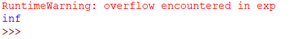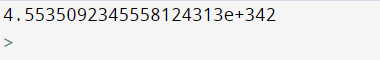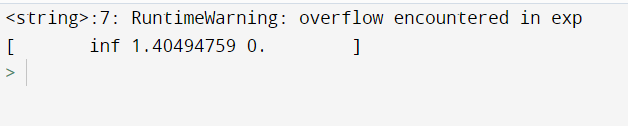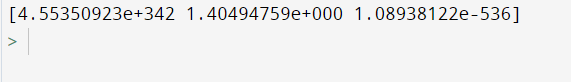Open in App
Not now

# How to Fix: RuntimeWarning: Overflow encountered in exp

• Difficulty Level : Basic
• Last Updated : 28 Nov, 2021

In this article we will discuss how to fix RuntimeWarning: overflow encountered in exp in Python.

This warning occurs while using the NumPy library’s exp() function upon using on a value that is too large. This function is used to calculate the exponential of all elements in the input array or an element (0-D Array of NumPy).

Example: Code to depict warning

## Python3

 `import` `numpy as np ` ` `  `print``(np.exp(``789``)) `

Output:The output is infinity cause e^789 is a very large value

This warning occurs because the maximum size of data that can be used in NumPy is float64 whose maximum range is 1.7976931348623157e+308. Upon taking logarithm its value becomes 709.782. For any larger value than this, the warning is generated.

Let us discuss ways to fix this.

## Method 1: Using float128

The data type float64 can be changed to float128.

Example: Program to fix the warning

## Python3

 `import` `numpy as np ` ` `  `x ``=` `789` `x ``=` `np.float128(x) ` `print``(np.exp(x)) `

Output:Using float128

For ndarray you can use the dtype parameter of the array method.

Example: Program to produce output without using dtype

## Python3

 `import` `numpy as np ` ` `  `cc ``=` `np.array([``789``, ``0.34``, ``-``1234.1``]) ` `print``(np.exp(cc)) `

Output:without using dtype

Example: Fixed warning by using dtype

## Python3

 `import` `numpy as np ` ` `  `cc ``=` `np.array([``789``, ``0.34``, ``-``1234.1``], dtype``=``np.float128) ` `print``(np.exp(cc)) `

Output:using dtype

## Method 2: Using filterwarnings()

Warning messages are typically issued in situations where it is useful to alert the user of some condition in a program, where that condition (normally) doesn’t warrant raising an exception and terminating the program. To deal with warnings there is a built-in module called warning. To read more about python warnings you can check out this article

The filterwarnings() function can be used to control the behavior of warnings in your programs. The warnings filter controls whether warnings are ignored, displayed, or turned into errors (raising an exception). This can be done using different actions:

• “ignore” to never print matching warnings
• “error” to turn matching warnings into exceptions
• “once” to print only the first occurrence of matching warnings, regardless of location

Syntax:

warnings.filterwarnings(action, message=”, category=Warning, module=”, lineno=0, append=False)

Example: Fixing warning using filterwarnings()

## Python3

 `import` `numpy as np ` `import` `warnings ` ` `  `# suppress warnings ` `warnings.filterwarnings(``'ignore'``) ` ` `  `x ``=` `789` `x ``=` `np.float128(x) ` `print``(np.exp(x)) `

Output:using filterwarnings()

My Personal Notes arrow_drop_up
Related Articles